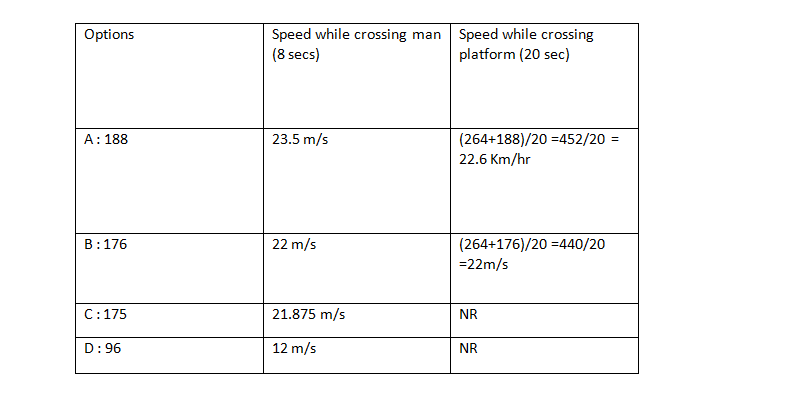• This is an assessment test.
• To draw maximum benefit, study the concepts for the topic concerned.
• Kindly take the tests in this series with a pre-defined schedule.

## Arithmetic: Time Speed Distance Test-10

Congratulations - you have completed Arithmetic: Time Speed Distance Test-10. You scored %%SCORE%% out of %%TOTAL%%. You correct answer percentage: %%PERCENTAGE%% . Your performance has been rated as %%RATING%%
 Question 1
A train passes a 50 metres long platform in 14 seconds and a man standing on the platform in 10 seconds. The speed of the train is:
 A 24 km/hr. B 36 km/hr. C 40 km/hr. D 45 km/hr.
Question 1 Explanation:
Let the length of train be L m.
L/10 = (L+50)/14
14L= 10L+500
L= 125 m .
Speed = (25/2) m/s = (25/2) x 18/5= 45 Km/hr.
 Question 2
A train passes a man standing on a platform in 8 seconds and also crosses the platform which is 264 metres long in 20 seconds. The length of the train (in metres) is:
 A 188 B 176 C 175 D 96
Question 2 Explanation:
Let the length of train be L m.
Acc. to question
(264+L)/20 = L/8
2112+8L=20L
L=  2112/12 = 176 m
 Question 3
A train moves past a telegraph post and a bridge 264 m long in 8 seconds and 20 seconds respectively. What is the speed of the train?
 A 69.5 km/hr. B 70 km/hr. C 79 km/hr. D 79.2 km/hr.
Question 3 Explanation:
Let the length of the train be L m.
L/8 = (264+L)/20
20L = 8L + 2112
L = 176 m.
Speed of train = 176/8 = 22 m/s =  22 X 18/5 = 79.2 Km/hr
 Question 4
A person standing on a railway platform noticed that a train took 21 seconds to completely pass through the platform which was 84 m long and it took 9 seconds in passing him. The speed of the train was
 A 25.2 km/hr. B 32.4 km/hr. C 50.4 km/hr. D 75.6 km/hr.
Question 4 Explanation:
Let the train’s length be L m.
L/9=(L+84)/21
21 L = 9L +756
12L= 756
L=63 m
Speed = 63 /9 = 7 m/s = 7 x 18/5 = 25.2 Km/hr.
 Question 5
A train passes a man standing on a platform in 8 seconds and also crosses the platform which is 264 metres long in 20 seconds. The length (in metres) of the train is
 A 188 B 176 C 175 D 96
Question 5 Explanation:
Analyzing by optionsClearly we can see that Option B is the answer.
Once you are finished, click the button below. Any items you have not completed will be marked incorrect.
There are 5 questions to complete.
 ← List →
Shaded items are complete.
 1 2 3 4 5 End